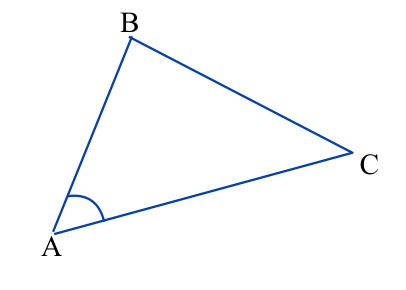## Congruent Triangles

This post about congruent triangles is part of a series of posts to help you prepare for the Advanced Algebra and Functions part of the Accuplacer test.

## Question

Angle A in triangle ABC is congruent to angle D in triangle DEF. Which of the statements below, if it’s true, proves that triangles ABC and DEF are congruent?

A.  and

B.   and

C.   and

D.   andTriangle DEF.Triangle ABC.

## Solution

Two triangles are congruent if one of these things is true:

• every side of one is congruent to a side of the other (SSS);
• two sides and the angle between them in one are congruent to two sides and the angle between them in the other (SAS); or
• two angles and any side in one are congruent to two angles and any side of the other (ASA or AAS).

Let’s go through the answer choices to see if one of them meets one of those criteria.Triangle DEF

A.  and .Triangle ABC

That’s  two  angles and the side between them in one triangle being congruent to the corresponding parts in the other triangle. That’s enough to meet the first criterion above, so it looks like that’s the answer. But let’s check the others.

B.   andTriangle ABC.

That’s two sides and an angle, but not the angle between them, so it does not do the job.Triangle DEF

C.   and .  Same problem as answer choice B: Two sides and one angle of one are congruent to two sides and one angle of the other, but the angle is not the one between the two sides, so that information is not enough for a proof.Triangle DEFTriangle ABC

D.  and . This gives you only congruent angles. Every proof of congruent triangles requires at least one side.Triangle ABC